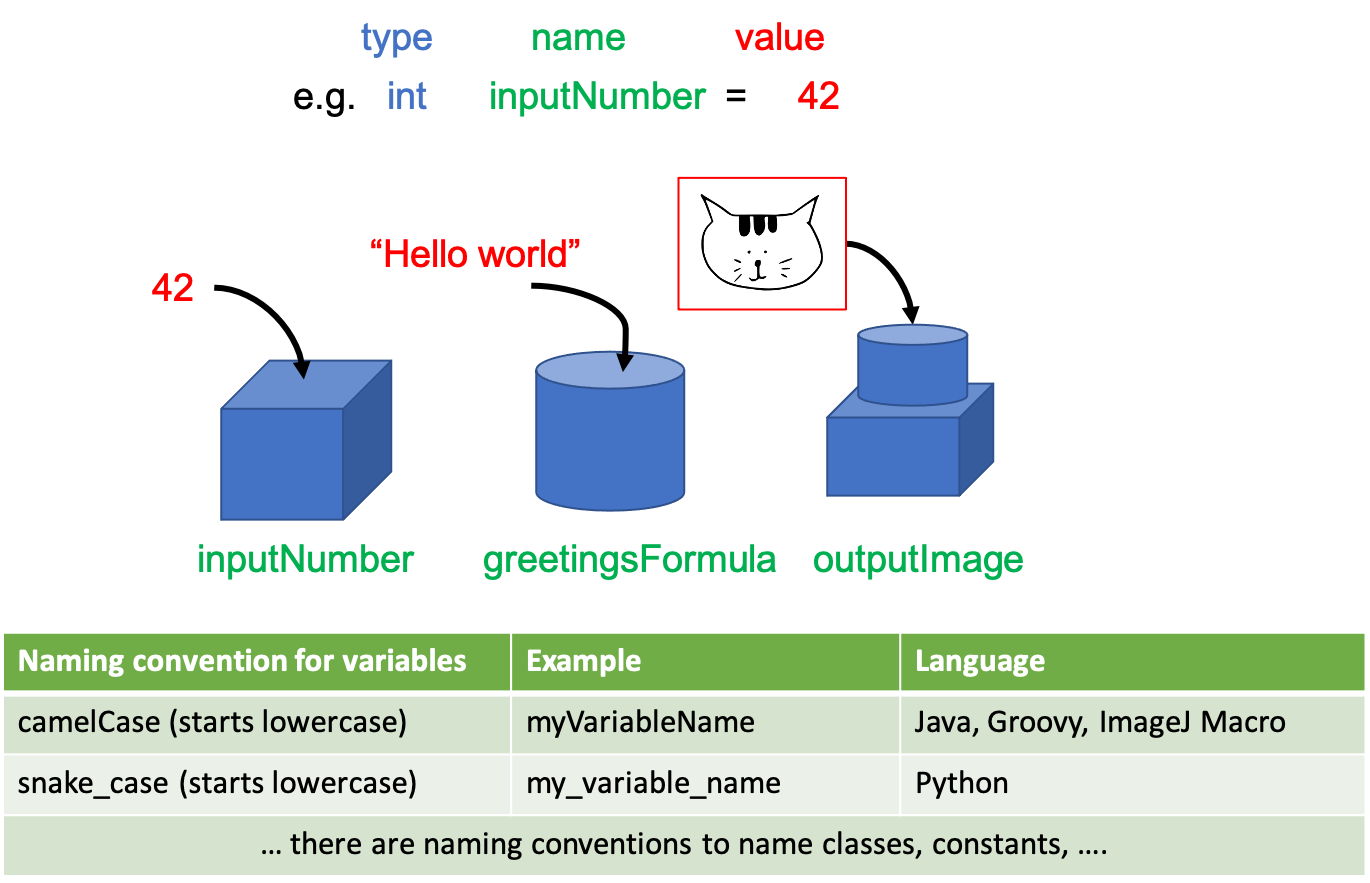## Prerequisites

Before starting this lesson, you should be familiar with:

## Learning Objectives

After completing this lesson, learners should be able to:
• Understand difference between variable name, value, type, and storage

• How to use variables in functions

## Motivation

A variable is an essential concept in programming, it allows to structure and generalize a script/program. A variable stores in memory a value, e.g. a numeric or string value, that can be used and changed at several occasions in a script.

## Concept map

graph TD V("Variable") --> |has|Name("Symbolic name") V --> |has|Type("Type") V --> |has|Value("Value") V --> |is| Stored("Stored in memory") Value --> |can| Change Type --> |can| Change

## FigureVariables are containers specific for an information type. Variable names do not contain spaces, should explain their purpose, should be consistent throughout your code, and should adhere to a naming convention.

## Activities

Use a script editor for all these activities.

### General usage of variables

• Show how variables need to be assigned/declared before usage
• Create two numeric variables and store results of addition in a new variable
• Use a numeric variable in a simple function (e.g. print(variable)) and in an image processing function

### String variables

• Show an example of a string variable and how to declare it

### Variable type

• Discuss variable type and, for some languages, type declaration
• Discuss possibility of type mismatch

Show activity for:

## Exercises

### Difference of Gaussians

The code performs two gaussian blurs of an image with fixed sigma. The code then computes the difference of the two filtered images.

1. Modify the code and replace the hard-coded sigma value of the gaussian blur with 2 variables, `sigma1` and `sigma2` respectively
2. Make `sigma2` three times larger than `sigma1` and run again the code

### Fix it

Try to run the code and fix the error(s). Modify the variable names to `camelCase` in a way that their content/meaning is clear to the reader.

Show exercise/solution for:

## ImageJ Macro, difference of gaussians

Copy the code to the FiJi script editor and solve the exercise.

Hint: You need to modify the code in line 5 and 7 and for example use the `&` operator to include the variables.

``````run("Close All")
open("https://github.com/NEUBIAS/training-resources/raw/master/image_data/xy_16bit__autophagosomes.tif");
rename("raw");
run("Duplicate...", "title=sigma1");
run("Gaussian Blur...", "sigma = 1");
run("Duplicate...", "title=sigma2");
run("Gaussian Blur...", "sigma = 1.5");
// This subtracts the blurred image from the raw image, i.e. sigma1 - sigma2
imageCalculator("Subtract create", "sigma1","sigma2");
``````

## Solution

``````run("Close All")
sigma1 = 1.0;
sigma2 = 1.5;
// For part two
// sigma2 = 3*sigma1;
open("https://github.com/NEUBIAS/training-resources/raw/master/image_data/xy_16bit__autophagosomes.tif");
rename("raw");
run("Duplicate...", "title=sigma1");
run("Gaussian Blur...", "sigma=&sigma1");
run("Duplicate...", "title=sigma2");
run("Gaussian Blur...", "sigma=&sigma2");
// This subtracts the blurred image from the raw image, i.e. sigma1 - sigma2
imageCalculator("Subtract create", "sigma1","sigma2");
``````

## ImageJ Macro, fix it

Copy the macro code to the FiJi editor and press `Run`. This will create an error.

1. Fix the variable naming for the file path. Use the `camelCase` naming convention.
2. Change the name of the variables `a` and `b` so that it their names are meaningful and reflect their content. Use the `camelCase` naming convention.
``````run("Close All");
FilePath = "https://github.com/NEUBIAS/training-resources/raw/master/image_data/xy_16bit__autophagosomes.tif";
a = 1;
b = 2;
open(filePath);
rename("input");
run("Duplicate...", "title=tophat");
selectWindow("input");
run("Duplicate...", "title=");
``````

## Solutions

``````run("Close All");
filePath = "https://github.com/NEUBIAS/training-resources/raw/master/image_data/xy_16bit__autophagosomes.tif";
open(filePath);
rename("input");
run("Duplicate...", "title=tophat");
selectWindow("input");
run("Duplicate...", "title=variance");
``````

## Assessment

### True or False

• A variable can only be a number
• Variables have a symbolic name that can be referenced to
• The value of a variable can’t change
• The name of a variable should indicate its content

• False
• True
• False
• True

## Follow-up material

Recommended follow-up modules: